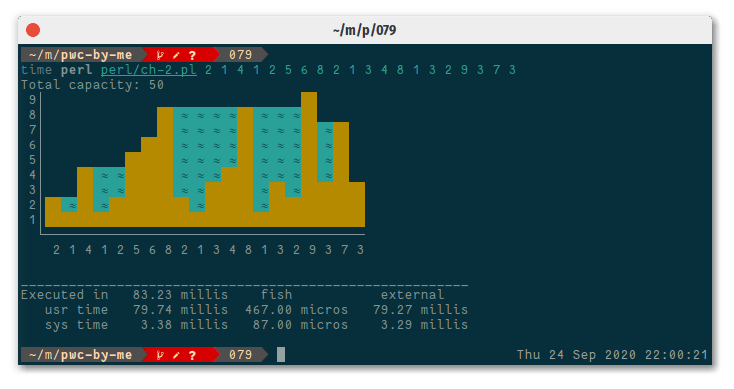## Advent Calendar - December 14, 2020

Monday, Dec 14, 2020| Tags: Perl### | Day 13 | Day 14 | Day 15 |

The gift is presented by Myoungjin Jeon. Today he is talking about his solution to the task `Trapped Rain Water` of “The Weekly Challenge - 079”. This is re-produced for Advent Calendar 2020 from the original post by Myoungjin Jeon.

It has been about 10 weeks since I joined the weekly challenge.

I’m not a professional programmer but I have experience with perl and some C lang. this is basically for perl/raku language but you can solve the many interesting challenges in “Other Language” - which is called “Guest” in their site - and have look into other’s code and enjoy the fantastic reviews as well.

I have learnt many things from others, some of them are still in mystery but it was such a great chance~!!

I will be probably too busy to code from next week and probably I cannot participate as I did in past weeks. I’d like to thank you them all and decided to post a blog about it.

so..

This is task #2 solution and there must be a wide range of solution method.

I’m starting from the left hand side data and have a look only right hand side and if there is any bigger (or equal) wall than left one.

I calculated the water capacity at every chance a possible reservoir can hold.

``````...

for ( my \$x = 1; \$x < @T; ) {
if ( \$left <= \$T[\$x] ) { # increasing only: no useful data
( \$start, \$left ) = ( \$x, \$T[\$x] );
++\$x;
}
elsif ( (my \$ri = firstidx { \$_ >= \$left } @T[\$x..\$#T]) >= 0 ) {
my \$abs_ri = \$x+\$ri;
my \$water_level = min( \$left, \$T[\$abs_ri] );
for ((\$start+1) .. (\$abs_ri-1)) { # water area only
\$W[\$_] = \$water_level - \$T[\$_]
}

( \$start, \$left ) = ( \$x, \$T[\$abs_ri] );
\$x += \$ri;
}

...
``````

but this approach have a problem at last section. I had to amend it by finding second tallest wall(which is smaller than left boundary though) and calculate the capacity if I had to.

``````    else { # generally decreasing ...
# find a tallest one as right boundary
my \$tallest = max @T[\$x .. \$#T];
if ( (my \$ri = firstidx { \$_ == \$tallest } @T[\$x..\$#T]) >= 0 ) {
my \$abs_ri = \$x+\$ri;
my \$water_level = min( \$left, \$T[\$abs_ri] );

for ((\$start+1) .. (\$abs_ri-1)) {
\$W[\$_] = \$water_level - \$T[\$_];
}
( \$start, \$left ) = ( \$x, \$T[\$x] );
++\$x;
}
}
...
``````

so if we sum @W array here we can get the total amount of capacity but this is not only thing we have to do.

we have to print Histogram!! I have some experience with printing on #75 so it wasn’t very hard. but the code itself is a bit long because I’d like to add some unicode character and color.

so this is how I look at if it is on my rxvt-unicodeand this is code if you want to look at

``````sub ssprintf (\$\$) { sprintf "%#\$_s", \$_ }
sub map_ssprintf  { map { sprintf "%#\$_s", \$_ } @_[1..\$#_] }

sub u_(\$) { # unicode
return \$_ unless \$::utf8;
my \$a = shift;
state %u = ( qw{` └
- ─
| │
~ ≈}, '#' => '■' );
\$u{\$a} // \$a
}

sub ch(\$\$;\$) { # unicode with colour
my \$a  = shift;
my \$ww = shift // 2;
my \$colour_str = shift;

if ( \$::colour and defined \$colour_str ) {
\$a eq '#' and \$a = ' '; # distinguish by color
return colored( [ \$colour_str ], ssprintf \$ww, u_\$a );
}
return ssprintf \$ww, u_\$a;
}

sub printTrappedInWater {
my @T = @{\$_}; # territory heights
my @W = @{\$_}; # water capacty

my \$maxh = max @T;                  # max height
my \$ww   = 1 + length \$maxh;        # word width

for my \$y ( reverse 1 .. \$maxh ) {
my \$line = ssprintf \$ww, \$y;
\$line .= u_"|";
for my \$x ( 0.. \$#T ) {
my \$ch;
if ( \$T[\$x] >= \$y ) {
\$ch = ch("#", \$ww, 'black on_yellow' );
}
elsif ( \$W[\$x] > 0 and \$T[\$x]+\$W[\$x] >= \$y ) {
\$ch = ch("~", \$ww, 'black on_cyan');
}
else {
\$ch = ch(" ", \$ww);
}
\$line .= \$ch;
}
say \$line;
}

say ssprintf( \$ww, " " ), u_"`",
map_ssprintf( \$ww, ( (u_("-") x \$ww ) x scalar @T ) );
say ssprintf(\$ww, " "), " ",map_ssprintf( \$ww, @T );
}
``````

so.. how do you think?

why don’t you join Perl Weekly Challenge?

If you have any suggestion then please do share with us perlweeklychallenge@yahoo.com.